Geometric Hales-Jewett Theorem (Solved)

 Importance: Medium ✭✭
 Author(s): Por, Attila Wood, David R.
 Subject: Geometry
 Keywords: Hales-Jewett Theorem ramsey theory
 Posted by: David Wood on: March 26th, 2014
 Solved by: Vytautas Gruslys. "A counterexample to a geometric Hales-Jewett type conjecture", J. Computational Geometry, 5.1:245-249, 2014.
Conjecture   For all integersand, there is an integersuch that for every setof at leastpoints in the plane, if each point inis assigned one ofcolours, then:
\itemcontainscollinear points, or \itemcontains a monochromatic line (that is, a maximal set of collinear points receiving the same colour)

This conjecture is trivially true for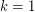with, and for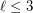and. The Motzkin-Rabin Theorem [PS] says that the conjecture is true for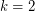with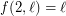. The conjecture is related to the Hales-Jewett Theorem, which states that for sufficiently large, every-colouring of the-dimensional grid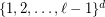contains a monochromatic "combinatorial"' line ofpoints. Say the Geometric Hales-Jewett Theorem is the statement obtained by replacing "combinatorial line" by "geometric line" (that is, a set of collinear points). This theorem is discussed in [P]. The conjecture is a generalisation of the Geometric Hales-Jewett Theorem.

This conjecture was disproved by Vytautas Gruslys [G].

Bibliography

[P] D.H.J. Polymath. Density Hales-Jewett and Moser numbers, arXiv:1002.0374, 2010.

*[PW] Attila Pór and David R. Wood. On visibility and blockers, J. Computational Geometry 1:29-40, 2010.

[PS] Lou M. Pretorius and Konrad J. Swanepoel. An Algorithmic Proof of the Motzkin-Rabin Theorem on Monochrome Lines, The American Mathematical Monthly 111.3:245-251, 2004.

[G] Vytautas Gruslys. A counterexample to a geometric Hales-Jewett type conjecture. J. Comput. Geom. 5.1:245-249, 2014.

* indicates original appearance(s) of problem.